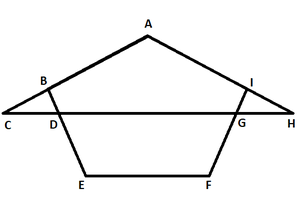# What is a common angle?

• Last Updated : 13 Dec, 2021

Geometry is one of the most historic parts of Mathematics. It’s an indispensable part of Ancient Mathematics. Geometry relates to the study of figures/line/points including different dimensions of figures/line/point such as measurement, position, size, surfaces, and shape. The different dimensions of the figure are studied and valid geometric rules are applied to study the relationship between different figures.

Geometry also deals with the different figures formed as solids 2-Dimensional, 3-Dimensional when lines meet at some inclination. The space is occupied by the figures (area), the boundary of the figures (perimeter), and the volume of the figures. An angle is formed when two lines meet at a point. The point of contact between the two lines is called the vertex. The degree of inclination between the two lines is a measure of their relationship.

### Types of angles

There are three types of angles possible based on the intersection of the lines. Then there is another classification based on triangles and the angles they form. Let’s learn about these classifications in detail,

Type of angles possible when two lines meet

1. Right Angle: An angle is said to be a right angle when the two lines meet perpendicularly i.e there are 90° with respect to each other.
2. Acute Angle: An angle is said to be an acute angle when the two lines meet between 0 to 90° with respect to each other.
3. Obtuse Angle: An angle is said to be an obtuse angle when the two lines meet between 90 to 180° with respect to each other.

Types of classification for triangles possible

• Right Angled Triangle: A triangle having one of the three angles as a right angle, falls under this category. Right angle triangle plays a very important role in trigonometry as well, Pythagoras theorem, etc. is derived for a right-angled triangle.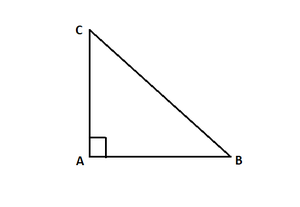Right- Angled Triangle

• Acute Angled Triangle: A triangle having all of the three angles as acute angles, falls under this category. In an acute-angled triangle, at least one of the two angles has to be obtuse (more than 90°).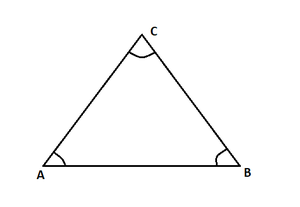Acute- Angled Triangle

• Obtuse Angled Triangle: A triangle having one of the three angles as an obtuse angle, falls under this category. In an obtuse angle, the rest of the two angles have to be acute (less than 90°) in nature.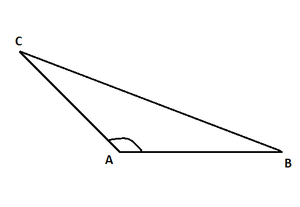Obtuse- Angled Triangle

### What is a common angle?

An angle is said to be common when the angle exists in more than one geometric figure at the same time. The common angle between two figures is nothing but two figures having common vertex point and angle edges. For instance, consider the below figure,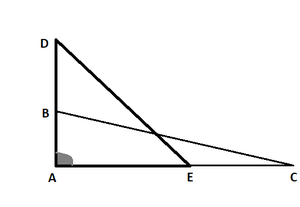Common ∠A

Here, in ABC and ADE, it is seen that ∠A is common in both triangles. ∠A is thus is the common angle for both the triangle figures. Another way of seeing a common angle in two figures is through the given observation: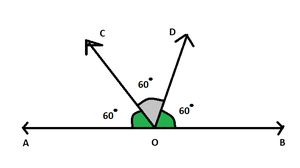Common Angle COD

Here, ∠AOD and ∠BOC are each of 120° formed as,

∠AOD = ∠AOC + ∠COD

∠BOC = ∠BOD + ∠COD

∠COD is the common angle between the two given angles. It is clearly evident from the figure as well, the blue shaded angle portion lies in both ∠AOD and ∠BOC.

### Sample Problems

Question 1: Find the common angle between the figures ABCD and AFG.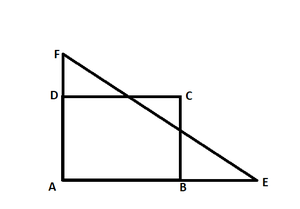The common angle between both the figures ABCD and AFG is angle A. Since ∠FAG and ∠DAB are the same in measurement and have common edges and common vertex A.

Question 2: Find the common angle between the figures ABC and CDE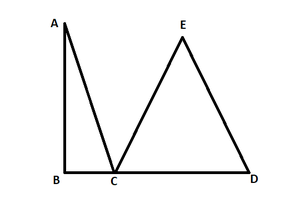There is no Common angle between the figures ABC and CDE as there is is no measurement of angle which is common to both the figures.

Question 3: Find the common angle between the figures ACH and ABEFI